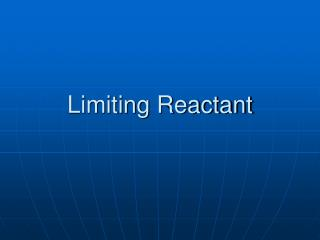DownloadDownload PresentationLimiting Reactant

# Limiting Reactant

Télécharger la présentation## Limiting Reactant

- - - - - - - - - - - - - - - - - - - - - - - - - - - E N D - - - - - - - - - - - - - - - - - - - - - - - - - - -
##### Presentation Transcript

1. Limiting Reactant

2. Which will run out first? • Each reactant’s amount will be provided, either in moles or mass • Two methods can be used • Trial Method • Advancement Method

3. Trial Method • Use mass-mass stoichiometry calculation to determine how much Reactant B will be required to completely consume Reactant A. • Sample Problem: 15.0g of N2 reacts with 15.0g of H2 to make ammonia according to the equation N2 + 3H2 → 2NH3 • How much ammonia can be made?

4. Solution to Ex. 1 • Trial method calculation • Advancement Method • Moles of each reactant (n)/coefficient then compare results • N2: 15.0g/28.02g/mol/1mol = 0.535 • H2: 15.0g/2.016g/mol/3mol = 2.48 • Lowest value is ALWAYS LR

5. And the point is… • Since it runs out first, the limiting reactant must be determined in order to determine the maximum theoretical yield for this reaction. • How much ammonia (NH3) can be made from these amounts of reactants? • Trial method calculation